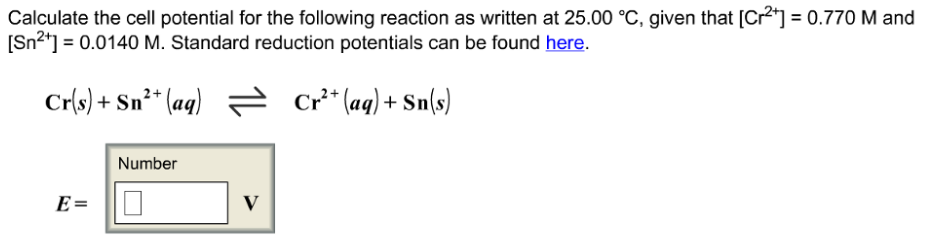# Problem: Calculate the cell potential for the following reaction as written at 25o C, given that [Cr2+]= 0.770 M and [Sn2+]= 0.0140 M.

###### FREE Expert Solution
81% (120 ratings)###### Problem Details

Calculate the cell potential for the following reaction as written at 25o C, given that [Cr2+]= 0.770 M and [Sn2+]= 0.0140 M.